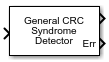# General CRC Syndrome Detector

Detect errors in received codeword frames according to generator polynomial

•Libraries:
Communications Toolbox / Error Detection and Correction / CRC

## Description

The General CRC Syndrome Detector block computes cyclic redundancy check (CRC) checksums for received codeword frames. For successful CRC detection in a communications system link, you must align the parameter settings of the General CRC Syndrome Detector block with the paired General CRC Generator block.

## Ports

### Input

expand all

Received codeword, specified as a binary column vector.

Data Types: `double` | `Boolean`

### Output

expand all

Output frame, returned as a binary column vector that inherits the data type of the input signal. The output frame contains the received codeword with the checksums removed.

The length of the output frame is n - k * r bits, where n is the size of the received codeword, k is the number of checksums per frame, and r is the degree of the generator polynomial.

Checksum error signal, returned as a binary column vector that inherits the data type of the input signal. The length of `Err` equals the value of Checksums per frame. For each checksum computation, an element value of 0 in `Err` indicates no checksum error, and an element value of 1 in `Err` indicates a checksum error.

## Parameters

expand all

Generator polynomial for the CRC algorithm, specified as one of the following:

• A polynomial character vector such as ```'z^3 + z^2 + 1'```.

• A binary row vector that represents the coefficients of the generator polynomial in order of descending power. The length of this vector is (N+1), where N is the degree of the generator polynomial. For example, ```[1 1 0 1]``` represents the polynomial x3+ z2+ 1.

• An integer row vector containing the exponents of z for the nonzero terms in the polynomial in descending order. For example, `[3 2 0]` represents the polynomial z3 + z2 + 1.

Some commonly used generator polynomials include:

CRC methodGenerator polynomial
CRC-32`'z^32 + z^26 + z^23 + z^22 + z^16 + z^12 + z^11 + z^10 + z^8 + z^7 + z^5 + z^4 + z^2 + z + 1'`
CRC-24 `'z^24 + z^23 + z^14 + z^12 + z^8 + 1'`
CRC-16 `'z^16 + z^15 + z^2 + 1'`
Reversed CRC-16`'z^16 + z^14 + z + 1'`
CRC-8`'z^8 + z^7 + z^6 + z^4 + z^2 + 1'`
CRC-4 `'z^4 + z^3 + z^2 + z + 1'`

Example: `'z^7 + z^2 + 1'`, ```[1 0 0 0 0 1 0 1]```, and `[7 2 0]` represent the same polynomial, p(z) = z 7 + z 2 + 1.

Initial states of the internal shift register, specified as a binary scalar or a binary row vector with a length equal to the degree of the generator polynomial. A scalar value is expanded to a row vector of equal length to the degree of the generator polynomial.

Select to use the direct algorithm for CRC checksum calculations. When cleared, the block uses the non-direct algorithm for CRC checksum calculations.

For more information on direct and non-direct algorithms, see Error Detection and Correction.

Select to flip the received codeword on a bytewise basis before entering the data into the shift register. When Reflect input bytes is selected, the received codeword length divided by the value of the Checksums per frame parameter must be an integer and a multiple of `8`. When Reflect input bytes is cleared, the block does not flip the input data.

Select Reflect checksums before final XOR to flip the CRC checksums around their centers after the input data are completely through the shift register. When Reflect checksums before final XOR is cleared, the block does not flip the CRC checksums.

Final XOR, specified as a binary scalar or a binary row vector with a length equal to the degree of the generator polynomial. The XOR operation runs using the value of the Final XOR parameter and the CRC checksum before comparing with the input checksum. A scalar value is expanded to a row vector of equal length to the degree of the generator polynomial. A setting of `0` is equivalent to no XOR operation.

Number of checksums calculated for each frame, specified as a positive integer.

## Block Characteristics

 Data Types `Boolean` | `double` Multidimensional Signals `no` Variable-Size Signals `yes`